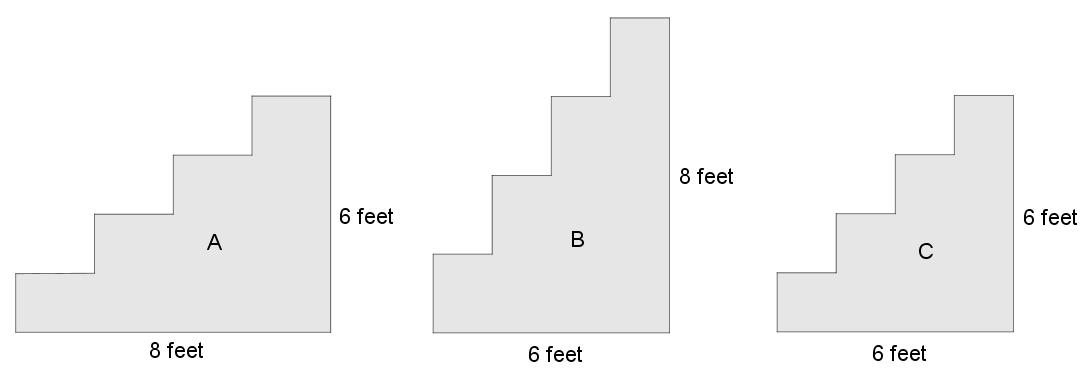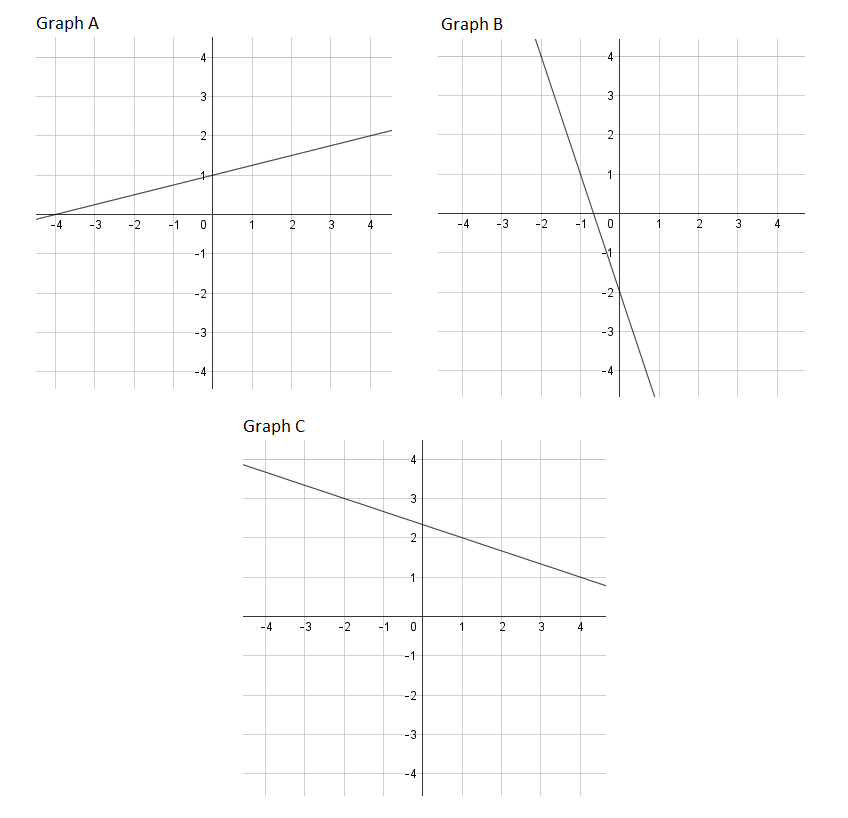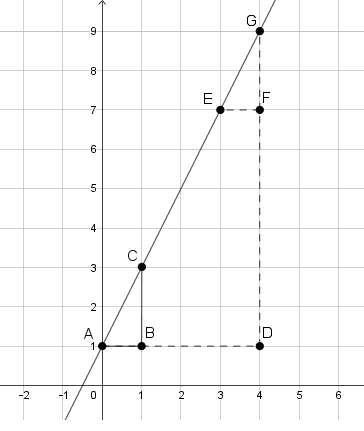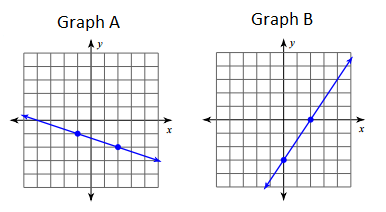# Linear Relationships

## Objective

Define slope and determine slope from graphs.

## Common Core Standards

### Core Standards

?

• 8.EE.B.6 — Use similar triangles to explain why the slope m is the same between any two distinct points on a non-vertical line in the coordinate plane; derive the equation y = mx for a line through the origin and the equation y = mx + b for a line intercepting the vertical axis at b.

?

• 8.G.A.4

• 8.G.A.5

• 7.NS.A.2.B

## Criteria for Success

?

1. Define the slope of a line as the measure of vertical change over the measure of horizontal change between any two points on the line.
2. Understand that the slope of a line is the same through any two points on the line, which can be explained using similar triangles.
3. Understand that positive slopes rise toward the right on the coordinate plane and negative slopes rise toward the left on the coordinate plane.
4. Understand that slopes with an absolute value measure less than 1 are more “shallow” and slopes with an absolute value measure greater than 1 are more “steep.”
5. Find the slope of a line from a graph.

## Tips for Teachers

?

• This lesson may be extended over two days in order to develop the concepts in all three Anchor Problems and to allow students time to practice on their own.
• Students will need an understanding that  ${-{{p}\over{q}}}$ is the same as ${-p\over{q}}$ and ${p\over{-q}}$ (standard 7.RP.2b) when they consider negative slope. It may be helpful to review this standard prior to this lesson.
• Students will also recall the transformations that can be used to show similarity between two figures (8.G.4).

#### Fishtank Plus

• Problem Set
• Student Handout Editor
• Vocabulary Package

## Anchor Problems

?

### Problem 1

Three staircases are shown below. Which staircase is the steepest? Without doing any calculations, order the staircases from least steep to most steep.Slope is a measure of steepness. The greater the slope, the greater the steepness. Use the measurements provided in the diagram to justify the order of steepness you determined earlier.

Another staircase climbs 8 feet over a distance of 10 feet. Where does this staircase fall in the order of steepness?

#### References

Finding Ways Staircase and Steepness

Staircase and Steepness by Fawn Nguyen is made available on Finding Ways under the CC BY 4.0 license. Accessed Feb. 24, 2018, 4:53 p.m..

Modified by The Match Foundation, Inc.

### Problem 2

Find the slope of the line in each graph below.### Problem 3

A line is drawn through the points A, C, E, and G as shown in the graph below.1. What transformation takes triangle $ABC$ to triangle $EFG$? Are the triangles congruent, similar, or neither? Explain.
2. What transformation takes triangle $ABC$ to triangle $ADG$? Are the triangles congruent, similar, or neither? Explain.
3. If two triangles are similar, then what do you know about their corresponding side lengths?
4. Will the slope of the line between any two points on the line always be the same? Explain your reasoning using similar triangles.

## Problem Set

?The following resources include problems and activities aligned to the objective of the lesson that can be used to create your own problem set.

?

1. Find the slope in each graph below.1. Use a different pair of coordinate points in Graph B to show the slope of the line is the same through any two points on the line.

#### References

Kuta Software Free Pre-Algebra Worksheets Finding SlopeProblem 1